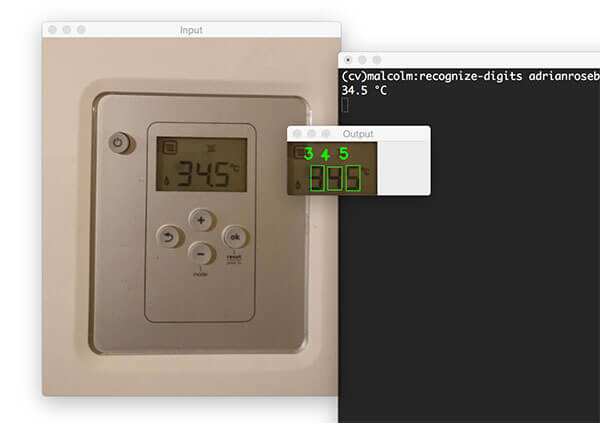# 七段显示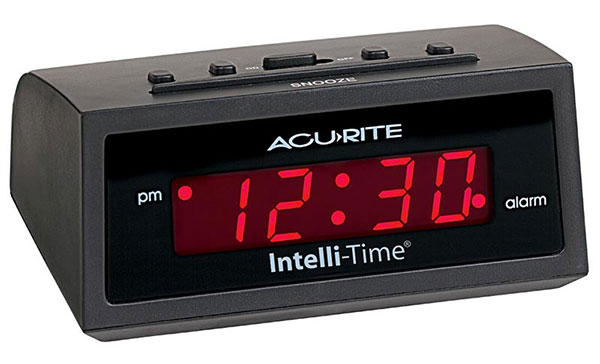闹钟上的每个数字都由一个七段组件表示，如下所示：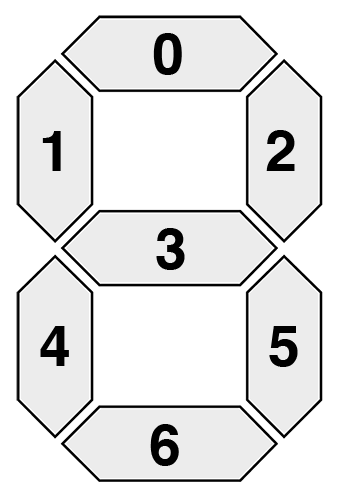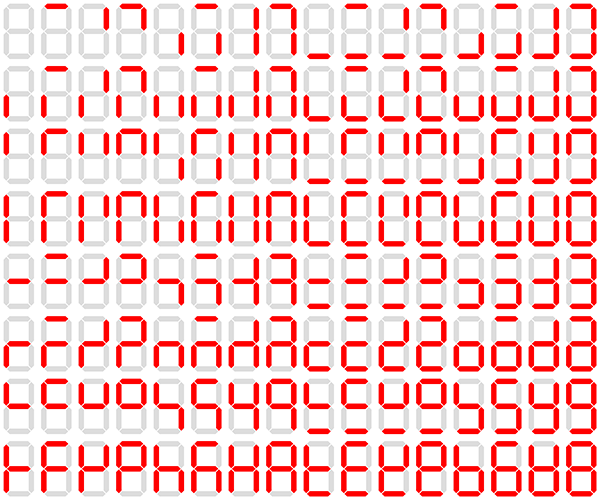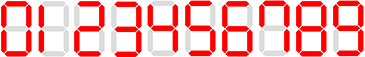# 设计OpenCV 数字识别器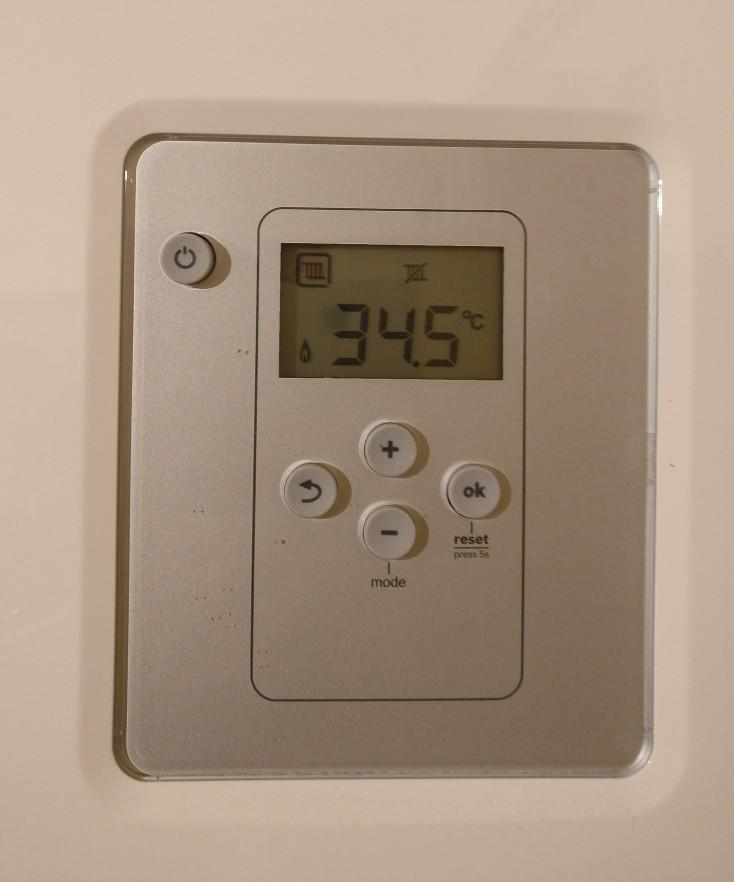```

# import the necessary packages

from imutils.perspective import four_point_transform from imutils import contours import imutils import cv2

# each digit on the thermostat

DIGITS_LOOKUP = { (1, 1, 1, 0, 1, 1, 1): 0, (0, 0, 1, 0, 0, 1, 0): 1, (1, 0, 1, 1, 1, 1, 0): 2, (1, 0, 1, 1, 0, 1, 1): 3, (0, 1, 1, 1, 0, 1, 0): 4, (1, 1, 0, 1, 0, 1, 1): 5, (1, 1, 0, 1, 1, 1, 1): 6, (1, 0, 1, 0, 0, 1, 0): 7, (1, 1, 1, 1, 1, 1, 1): 8, (1, 1, 1, 1, 0, 1, 1): 9 } ```

`pip install imutils`

```

# graycale, blurring it, and computing an edge map

image = imutils.resize(image, height=500) gray = cv2.cvtColor(image, cv2.COLOR_BGR2GRAY) blurred = cv2.GaussianBlur(gray, (5, 5), 0) edged = cv2.Canny(blurred, 50, 200, 255) ```

• 调整大小。
• 将图像转换为灰度。
• 使用 5×5 内核应用高斯模糊以减少高频噪声。
• 通过 Canny 边缘检测器计算边缘图。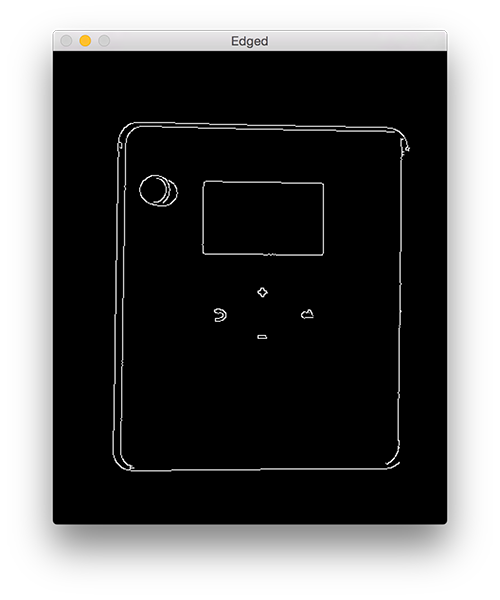注意 LCD 的轮廓是如何清晰可见的——这完成了步骤 #1。 我们现在可以继续第 2 步，提取 LCD 本身：

```

# size in descending order

cnts = cv2.findContours(edged.copy(), cv2.RETR_EXTERNAL, cv2.CHAIN_APPROX_SIMPLE) cnts = imutils.grab_contours(cnts) cnts = sorted(cnts, key=cv2.contourArea, reverse=True) displayCnt = None

# loop over the contours

for c in cnts: # approximate the contour peri = cv2.arcLength(c, True) approx = cv2.approxPolyDP(c, 0.02 * peri, True) # if the contour has four vertices, then we have found # the thermostat display if len(approx) == 4: displayCnt = approx break ```

```

# to it

warped = four_point_transform(gray, displayCnt.reshape(4, 2)) output = four_point_transform(image, displayCnt.reshape(4, 2)) ```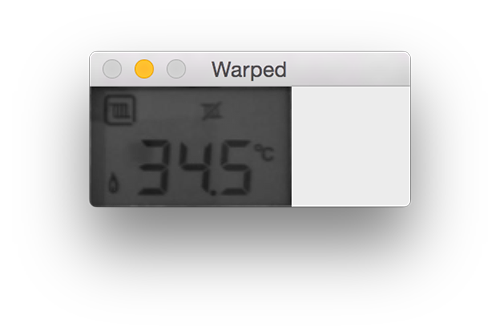```

# operations to cleanup the thresholded image

thresh = cv2.threshold(warped, 0, 255, cv2.THRESH_BINARY_INV | cv2.THRESH_OTSU) kernel = cv2.getStructuringElement(cv2.MORPH_ELLIPSE, (1, 5)) thresh = cv2.morphologyEx(thresh, cv2.MORPH_OPEN, kernel) ```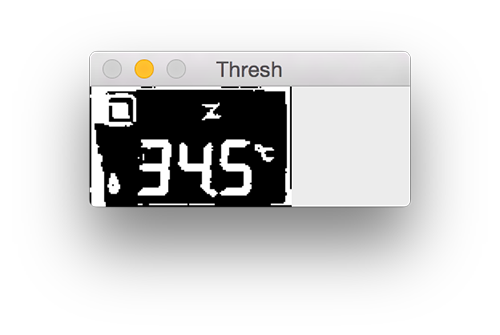然后我们应用一系列形态学操作来清理阈值图像：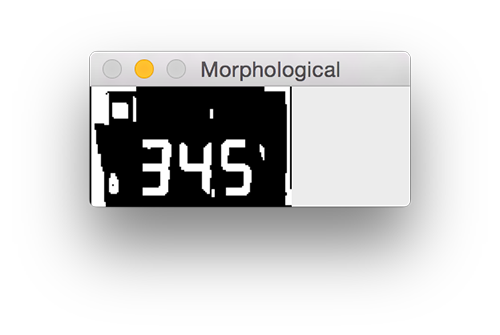```

# digit contours lists

cnts = cv2.findContours(thresh.copy(), cv2.RETR_EXTERNAL, cv2.CHAIN_APPROX_SIMPLE) cnts = imutils.grab_contours(cnts) digitCnts = []

# loop over the digit area candidates

for c in cnts: # compute the bounding box of the contour (x, y, w, h) = cv2.boundingRect(c) # if the contour is sufficiently large, it must be a digit if w >= 15 and (h >= 30 and h <= 40): digitCnts.append(c) ```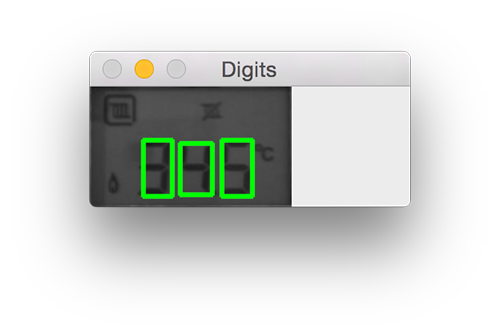```

# actual digits themselves

digitCnts = contours.sort_contours(digitCnts, method="left-to-right") digits = [] ```

```

# loop over each of the digits

for c in digitCnts: # extract the digit ROI (x, y, w, h) = cv2.boundingRect(c) roi = thresh[y:y + h, x:x + w] # compute the width and height of each of the 7 segments # we are going to examine (roiH, roiW) = roi.shape (dW, dH) = (int(roiW * 0.25), int(roiH * 0.15)) dHC = int(roiH * 0.05) # define the set of 7 segments segments = [ ((0, 0), (w, dH)), # top ((0, 0), (dW, h // 2)), # top-left ((w - dW, 0), (w, h // 2)), # top-right ((0, (h // 2) - dHC) , (w, (h // 2) + dHC)), # center ((0, h // 2), (dW, h)), # bottom-left ((w - dW, h // 2), (w, h)), # bottom-right ((0, h - dH), (w, h)) # bottom ] on =  * len(segments) ```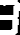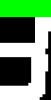```

# loop over the segments

``````for (i, ((xA, yA), (xB, yB))) in enumerate(segments):
# extract the segment ROI, count the total number of
# thresholded pixels in the segment, and then compute
# the area of the segment
segROI = roi[yA:yB, xA:xB]
total = cv2.countNonZero(segROI)
area = (xB - xA) * (yB - yA)
# if the total number of non-zero pixels is greater than
# 50% of the area, mark the segment as "on"
if total / float(area) > 0.5:
on[i]= 1
# lookup the digit and draw it on the image
digit = DIGITS_LOOKUP[tuple(on)]
digits.append(digit)
cv2.rectangle(output, (x, y), (x + w, y + h), (0, 255, 0), 1)
cv2.putText(output, str(digit), (x - 10, y - 10),
cv2.FONT_HERSHEY_SIMPLEX, 0.65, (0, 255, 0), 2)
``````

```

```

# display the digits

print(u"{}{}.{} \u00b0C".format(*digits)) cv2.imshow("Input", image) cv2.imshow("Output", output) cv2.waitKey(0) ```